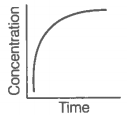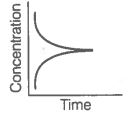Select Chapter Topics:Match the following equilibria with the corresponding condition.

 A. Liquid$⇌$Vapour 1. Saturated solution B. Solid$⇌$Liquid 2. Boiling point C. Solid$⇌$Vapour 3. Sublimation point D. Solute (s)$⇌$Solute (solution) 4. Melting point 5. Unsaturated solution

Codes

 A B C D 1. 2 4 3 1 2. 1 2 3 5 3. 5 4 3 2 4. 4 5 3 2Subtopic:  Introduction To Equilibrium |
88%
From NCERT
To view explanation, please take trial in the course.
NEET 2023 - Target Batch - Aryan Raj Singh
Hints
To view explanation, please take trial in the course.
NEET 2023 - Target Batch - Aryan Raj Singh

For the reaction : N2(g) + 3H2(g) →2NH3(g)
$$\text { Equilibrium constant } K_{c}=\frac{\left[\mathrm{NH}_{3}\right]^{2}}{\left[\mathrm{~N}_{2}\right]\left[\mathrm{H}_{2}\right]^{3}}$$
Some reactions are written below in Column I
and their equilibrium constants
in terms of Kc are written in Column II.
Match the following reactions with the corresponding equilibrium constant.

 Column I (Reaction) Column II (Equilibrium constant) A. $2{\mathrm{N}}_{2}\left(\mathrm{g}\right)+6{\mathrm{H}}_{2}\left(\mathrm{g}\right)⇌4{\mathrm{NH}}_{3}\left(\mathrm{g}\right)$ 1. $2{\mathrm{K}}_{\mathrm{c}}$ B. $2{\mathrm{NH}}_{3}\left(\mathrm{g}\right)⇌{\mathrm{N}}_{2}\left(\mathrm{g}\right)+3{\mathrm{H}}_{2}\left(\mathrm{g}\right)$ 2. ${\mathrm{K}}_{\mathrm{c}}^{1/2}$ C. $\frac{1}{2}{\mathrm{N}}_{2}\left(\mathrm{g}\right)+\frac{3}{2}{\mathrm{H}}_{2}\left(\mathrm{g}\right)⇌{\mathrm{NH}}_{3}\left(\mathrm{g}\right)$ 3. $\frac{1}{{\mathrm{K}}_{\mathrm{c}}}$ 4. ${\mathrm{K}}_{\mathrm{c}}^{2}$

Codes

 A B C 1. 4 3 2 2. 1 2 3 3. 1 4 3 4. 4 1 3Subtopic:  Kp, Kc & Factors Affecting them |
87%
From NCERT
To view explanation, please take trial in the course.
NEET 2023 - Target Batch - Aryan Raj Singh
Hints
To view explanation, please take trial in the course.
NEET 2023 - Target Batch - Aryan Raj Singh

Match the standard free energy of the reaction with the corresponding equilibrium constant.

 A. $∆{\mathrm{G}}^{⊝}>0$ 1. K>1 B. $∆{\mathrm{G}}^{⊝}<0$ 2. K=1 C. $∆{\mathrm{G}}^{⊝}=0$ 3. K=0 4. K<1

Codes

 A B C 1. 4 1 2 2. 1 2 3 3. 2 4 3 4. 4 1 3Subtopic:  Kp, Kc & Factors Affecting them |
66%
From NCERT
To view explanation, please take trial in the course.
NEET 2023 - Target Batch - Aryan Raj Singh
Hints
To view explanation, please take trial in the course.
NEET 2023 - Target Batch - Aryan Raj Singh

Match the following species with the corresponding conjugate acid.

 Species Conjugate acid A.  $N{H}_{3}$ 1. $C{O}_{3}^{2-}$ B. $HC{O}_{3}^{-}$ 2. $N{H}_{4}^{+}$ C. ${H}_{2}O$ 3.${H}_{3}{O}^{+}$ D. $HS{O}_{4}^{-}$ 4. ${H}_{2}C{O}_{3}$ 5. ${H}_{2}S{O}_{4}$

Codes

 A B C D 1. 2 5 1 5 2. 2 4 3 5 3. 5 4 3 2 4. 4 5 3 2Subtopic:  Acids & Bases - Definitions & Classification |
94%
From NCERT
To view explanation, please take trial in the course.
NEET 2023 - Target Batch - Aryan Raj Singh
Hints
To view explanation, please take trial in the course.
NEET 2023 - Target Batch - Aryan Raj Singh

 A B A.i. Variation in product concentration with time. B.ii. Reaction at equilibrium C.iii. Variation in reactant concentration with time.

Match the graphical variations with their descriptions given above and identify the correct codes below:

 A B C 1. i iii ii 2. i ii iii 3. iii ii i 4. iii i iiSubtopic:  Kp, Kc & Factors Affecting them |
70%
From NCERT
To view explanation, please take trial in the course.
NEET 2023 - Target Batch - Aryan Raj Singh
Hints
To view explanation, please take trial in the course.
NEET 2023 - Target Batch - Aryan Raj Singh

Match the Column I with Column II

 Column I Column II A. Equilibrium 1. B. Spontaneous  reaction 2. $∆\mathrm{G}=0$ C. Non-spontaneous reaction 3.

Codes

 Options: A B C 1. 2 3 1 2. 1 2 3 3. 2 1 3 4. 3 1 2Subtopic:  Kp, Kc & Factors Affecting them |
82%
From NCERT
To view explanation, please take trial in the course.
NEET 2023 - Target Batch - Aryan Raj Singh
Hints
To view explanation, please take trial in the course.
NEET 2023 - Target Batch - Aryan Raj Singh

Given below are two statements:

 Assertion (A): The increasing order or acidity of hydrogen halides is HF

 1 Both (A) and (R) are true and (R) is the correct explanation of (A). 2 Both (A) and (R) are true but (R) is not the correct explanation of (A). 3 (A) is true but (R) is false. 4 (A) is false but (R) is true.Subtopic:  Acids & Bases - Definitions & Classification |
81%
From NCERT
To view explanation, please take trial in the course.
NEET 2023 - Target Batch - Aryan Raj Singh
Hints
To view explanation, please take trial in the course.
NEET 2023 - Target Batch - Aryan Raj Singh

Given below are two statements:

 Assertion (A): A solution containing a mixture of acetic acid and sodium acetate maintains a constant value of pH on the addition of small amounts of acid or alkali. Reason (R): A solution containing a mixture of acetic acid and sodium acetate acts as a buffer solution.

 1 Both (A) and (R) are true and (R) is the correct explanation of (A). 2 Both (A) and (R) are true but (R) is not the correct explanation of (A). 3 (A) is true but (R) is false. 4 (A) is false but (R) is true.Subtopic:  Buffer |
89%
From NCERT
To view explanation, please take trial in the course.
NEET 2023 - Target Batch - Aryan Raj Singh
Hints
To view explanation, please take trial in the course.
NEET 2023 - Target Batch - Aryan Raj Singh

Given below are two statements:

 Assertion (A): The ionisation of hydrogen sulphide in water is low in the presence of hydrochloric acid Reason (R): Hydrogen sulphide is a weak acid.

 1 Both (A) and (R) are true and (R) is the correct explanation of (A). 2 Both (A) and (R) are true but (R) is not the correct explanation of (A). 3 (A) is true but (R) is false. 4 (A) is false but (R) is true.Subtopic:  Common Ion Effect |
51%
From NCERT
To view explanation, please take trial in the course.
NEET 2023 - Target Batch - Aryan Raj Singh
Hints
To view explanation, please take trial in the course.
NEET 2023 - Target Batch - Aryan Raj Singh

 Assertion (A): For any chemical reaction at a particular temperature, the equilibrium constant is fixed and is a characteristic property. Reason (R): Equilibrium constant is independent of temperature.

 1 Both (A) and (R) are true and (R) is the correct explanation of (A). 2 Both (A) and (R) are true but (R) is not the correct explanation of (A). 3 (A) is true but (R) is false. 4 (A) is false but (R) is true.Subtopic:  Kp, Kc & Factors Affecting them |
84%
From NCERT
To view explanation, please take trial in the course.
NEET 2023 - Target Batch - Aryan Raj Singh
Hints
To view explanation, please take trial in the course.
NEET 2023 - Target Batch - Aryan Raj Singh

Select Chapter Topics: